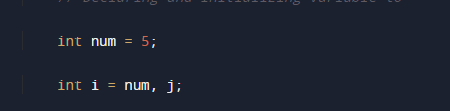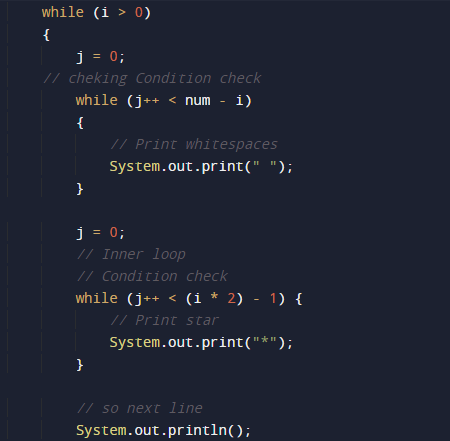# Java Program to Print Invert Triangle

In this tutorial you will learn about the Java Program to Print Invert Triangle and its application with practical example.

In this tutorial, we will learn to create a Java program that will Print Inverted Triangle using Java programming Language.

## Prerequisites

Before starting with this tutorial we assume that you are best aware of the following Java programming topics:

• Java Operators.
• Basic Input and Output
• Class and Object.
• Basic Java programming.
• Loops in Java.
• Nested for loop in Java.

## Invert Triangle of Star

To print Inverted Triangle Star patterns in Java , we will use nested for loops. The outermost loop is responsible for the number of rows in star (*) of Inverted Triangle,whereas inner one  loop will print the required number of stars * in each row.## Java Program to Print Invert Triangle

In this program we will print Inverted Triangle of Star using nested “For” loop and a while loop. We would first declared and initialized the required variables. Then we will create the “Inverted Triangle” of Star using below program.Let’s have a look.

## Output

Java Program to Print Invert TriangleIn the above program, we have first declared and initialized a set variables required in the program.

• num = it will hold size or pyramid
• i and j= it will use to iterate loops.Now we going  to run loops

Outermost loop to iterate through a number of rows as initialize . Now,Run an inner loop from 1 to ‘i-1’

As you can see in the image above ,and again run another inner loop from 1 to rows * 2 – (i × 2 – 1) that will print over desire output.We had printed reverse pyramid star(*) pattern using the following methods.

In this tutorial we have learn about the Java Program to Print Invert Triangle and its application with practical example. I hope you will like this tutorial.